## Session 11: Introduction to machine learning¶

Most of the code is directly adapted from the examples of Andrej Karpathy's CS231n course (available $\href{http://cs231n.github.io/neural-networks-case-study/}{here}$ and $\href{https://github.com/bagavi/CS231N/tree/master/assignment1}{there}$).

Author : Gwendoline De Bie (text by Jean Feydy)

Vocabulary. We consider the general setting of supervised learning: given a set of observations $(x_i, y_i)$, we would like to learn a $w$-parameterized model $f_w$ such that $f_w(x_i) \approx y_i$: the $x_i$'s are the data points, and the $y_i$'s are the labels. If the $y_i$'s were continuous function values, we would be tackling a regression problem; but today, we're interested in a discrete classification problem were the $y_i$'s are labels living in an arbitrary set $\{\text{blue, yellow, red}\}$ identified for mathematical convenience with the integer set $\{1,2,3\}$ - or $\{0,1,2\}$ to fit python's indexing conventions.

Notations. In the equations written below, $x_i\in \mathbb{R}^2$ and $y_i\in \{1, \dots, K\}=\{1,2,3\}$ will denote generic data points, $x$ and $y$ will be generic variables of deterministic functions, whereas the capital letters $X$ and $Y$ denote the observed training samples $(x_i)_{1\leqslant i\leqslant I}$ and $(y_i)_{1\leqslant i\leqslant I}$.

The probabilistic hypothesis here is that the $x_i$ are independent identically distributed variables, with $y_i$ following a law $g_{x_i}$ that we wish to regress. At the very least, we would like to be able to compute the maximum likelihood estimator

$$\arg\max_y g_{x_i}(y),~~~~ \text{ approximated by the parametric function }~~~~ f_w(x_i)$$

and then assume a "signal+noise" generative process: $y_i \sim g_{x_i} \simeq \mathcal{N}(f_w(x_i), \sigma^2)$ for instance.

The parameters $w$ of the model are learnt through the minimization of a loss function of the form

$$\text{Cost}_{X,Y}(w) ~=~ \sum_{i=1}^I \text{Att}(f_w(x_i), y_i) + \text{Reg}(w).$$

The optimal set of parameters minimizes the sum of a data attachment term $\sum_i\text{Att}(f_w(x_i), y_i)$ and of an explicit regularization term $\text{Reg}(w)$. Now, remark that this problem it is equivalent to the maximization of

$$\exp\big(-\text{Cost}_{X,Y}(w)\big) ~=~ \exp\big(-\text{Reg}(w)\big) \cdot\prod_i \exp\big(-\text{Att}(f_w(x_i), y_i)\big)$$

which can be written, up to a multiplicative renormalization constant, as a conditional likelihood

$$\mathbb{P}(w,Y ~|~ X) ~=~ \mathbb{P}(w) ~\cdot~ \prod_i \mathbb{P}(y_i ~|~ w, x_i).$$

If both $\text{Reg}$ and $\text{Att}$ are quadratic functions, minimizing $\text{Cost}$ is akin to computing a Maximum A Posteriori estimation of $w$ for a Gaussian generative model, which explains the empirical data distribution as follow:

• The input data $X$ is a deterministic input.
• The model's parameters $w$ are drawn independently of $X$, according to a fixed Gaussian distribution.
• The "clean" values $f_w(x_i)$ are computed independently from each other, before corruption by an additive Gaussian noise results in the observed values $y_i$.
In :
# A bit of setup
import numpy as np
import matplotlib.pyplot as plt
from sklearn import svm
from sklearn.datasets import fetch_mldata
from sklearn.linear_model import LogisticRegression
from sklearn.model_selection import train_test_split
from sklearn.preprocessing import StandardScaler
import time

%matplotlib inline
#plt.rcParams['figure.figsize'] = (10.0, 8.0) # set default size of plots
plt.rcParams['image.interpolation'] = 'nearest'
plt.rcParams['image.cmap'] = 'gray'



### Generating some (not easily linearly separable) data¶

We generate 2D synthetic data to be classified, preferably not easily linearly separable so that it's a bit more exciting and that more elaborate models appear relevant to the classification task.

In :
np.random.seed(0)
N = 100 # number of points per class
D = 2   # dimensionality
K = 3   # number of classes
I = N*K # Total number of points
X = np.zeros((N*K,D))
y = np.zeros(N*K, dtype='uint8')
for j in range(K):
ix = range(N*j,N*(j+1))
t = np.linspace(j*4,(j+1)*4,N) + np.random.randn(N)*0.2 # theta
X[ix] = np.c_[r*np.sin(t), r*np.cos(t)]
y[ix] = j
Y = np.atleast_2d(y).T.astype(float) # Vector made out of y, for convenience
fig = plt.figure()
plt.scatter(X[:, 0], X[:, 1], c=y, s=40, cmap=plt.cm.Spectral)
plt.axis('equal') ; plt.xlim([-1,1]) ; plt.ylim([-1,1]) ;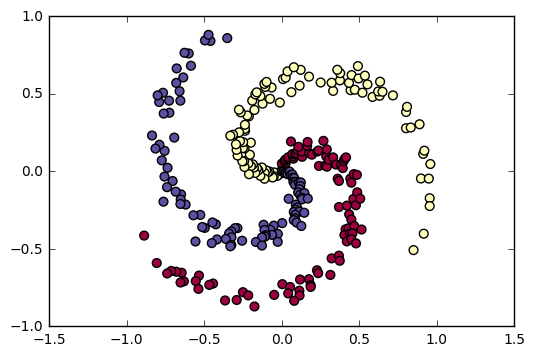## A simplistic linear regression¶

The simplest model of them all is to view $y_i$ as a real-valued function of $x_i$, and to fit a linear model of parameters $W \in \mathbb{R}^2, b \in \mathbb{R}$ by using

$$f_{W,b}(x) ~=~ \langle W, x \rangle + b$$

with no regularization and an $L^2$ attachment term (linked to a Gaussian noise hypothesis). That is, we minimize

$$\text{Cost}_{X,Y}(W,b) ~=~ \frac{1}{2 I}\sum_i \| f_{W,b}(x_i) - y_i \|^2.$$

Now, this minimization problem can be solved explicitely using the pseudoinverse... But for the sake of it, let's use gradient descent instead!

In :
# initialize parameters randomly
W = np.random.randn(2,1) # Python conventions : vectors in line, hence linear forms in column
b = 0

# some hyperparameters
step_size = 1e-0

num_examples = X.shape
for i in range(201):
scores = np.dot(X, W) + b
loss   = (.5/I) * np.sum( (scores - Y)**2 )
if i % 20 == 0:
print("iteration %d: loss %f" % (i, loss))

# compute the gradient on scores
dscores = (scores - Y) / I

# backpropate the gradient to the parameters (W,b)
dW = np.dot(X.T, dscores)
db = np.sum(dscores)

# perform a parameter update
W += -step_size * dW
b += -step_size * db

iteration 0: loss 0.911575
iteration 20: loss 0.265345
iteration 40: loss 0.265204
iteration 60: loss 0.265204
iteration 80: loss 0.265204
iteration 100: loss 0.265204
iteration 120: loss 0.265204
iteration 140: loss 0.265204
iteration 160: loss 0.265204
iteration 180: loss 0.265204
iteration 200: loss 0.265204

In :
# evaluate training set accuracy
scores = np.dot(X, W) + b
predicted_class = np.maximum(np.minimum(np.round(scores), 2), 0)
print('training accuracy: %.2f' % (np.mean(predicted_class == Y)))

training accuracy: 0.44

In :
# plot the resulting classifier
h = 0.02
x_min, x_max = X[:, 0].min() - 1, X[:, 0].max() + 1
y_min, y_max = X[:, 1].min() - 1, X[:, 1].max() + 1
xx, yy = np.meshgrid(np.arange(x_min, x_max, h),
np.arange(y_min, y_max, h))
Z = np.dot(np.c_[xx.ravel(), yy.ravel()], W) + b
Z = np.maximum(np.minimum(np.round(Z), 2), 0)
Z = Z.reshape(xx.shape)
fig = plt.figure()
plt.contourf(xx, yy, Z, cmap=plt.cm.Spectral, alpha=0.8)
plt.scatter(X[:, 0], X[:, 1], c=y, s=40, cmap=plt.cm.Spectral)
plt.axis("equal") ; plt.xlim(xx.min(), xx.max()) ; plt.ylim(yy.min(), yy.max())

Out:
(-1.8712034092398278, 1.8687965907601756)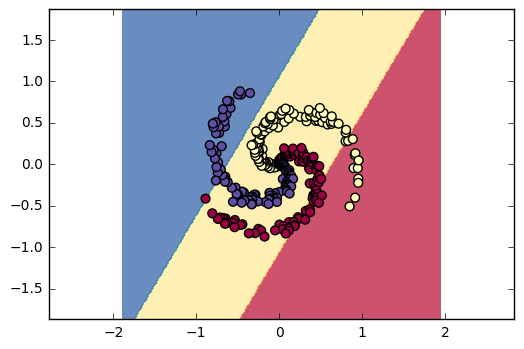Well. Looks like we'll have to be a little bit more clever ;-)

## Training a softmax linear classifier¶

Contrary to the standard linear model (that treats the class label $y(x)$ as a real-valued function), a softmax linear classifier allows us to handle labels as discrete variables. We still compute linear scores, but now interpret them as the unnormalized log-probabilities of each class, without relying on any arbitrary ordering of the labels.

If $K$ is the number of class, the trainable parameters of our model are a weight matrix $W$ of size $K\times D$ (in the math convention, where vectors are columns... and not lines, as in Python), and an offset vector $B$ of size $K$. The linear scores $s\in \mathbb{R}^K$ at a generic location $x \in \mathbb{R}^D$ are computed as

$$s ~=~ W x + b$$

and we decide to interpret those generic "class scores" as un-normalized log probabilities. That is, given $s = (s, \dots, s[K])$ a given score vector, we assume that

$$P_s[k] ~=~ \mathbb{P}(\text{class} = k ~|~ s) ~=~ \frac{ \exp(s[k]) }{\sum_{j=1}^K \exp(s[j])}.$$

$f_w(x_i)$ is therefore best interpreted as a probability measure on the discrete space of labels $\{1,2,\dots, K\}$. The exponential in the formula above is convenient, because it allows us to represent with scores that a relatively close to each other a wide range of positive probabilities.

Notice how we passed from scalar-valued labels to probabilities on the set of labels. It is clever: as there is no satisfying way of defining a distance in the naive space of labels, we embed our feature space in a larger set (that of probability measures), in which standard discrepancies/divergences will define suitable data attachment formulas.

Now, what kind of data attachment term $\text{Att}(f_w(x_i), y_i)$ could we use to compare the "generated" $f_w(x_i)\simeq P_{Wx_i+b}$ to the "deterministic" empiric law $$y_i ~~ \text{identified with the measure} ~~ \delta_{y_i} ~~ \text{such that} ~~ \mathbb{P}_{\delta_{y_i}}(\text{class} = k) = \begin{cases}1 & \text{ if } k = y_i \\ 0 & \text{otherwise}\end{cases}~~~~ ?$$ Given that $\{1,2,\dots, K\}$ is a label space without any relevant metric structure, a good choice is the cross-entropy or Kullback-Leibler divergence

$$KL( \delta_y ~||~ P_s ) ~=~ \int \log \bigg( \frac{\text{d}\delta_y}{\text{d}P_s}\bigg) ~ \text{d}\delta_y ~=~ -\log(P_s[y]) ~=~ - \log \bigg( \frac{ \exp(s[y]) }{\sum_{j=1}^K \exp(s[j])} \bigg) ~~~~~ \text{since } \delta_y \text{ is completely localized on} ~y.$$

Remember from the course on information theory and entropic coding that the KL-divergence, or cross-entropy between two probability measures $\mu$ and $\nu$, is given by

$$KL( \mu~||~\nu) ~=~ \text{«} \underbrace{\int \log\bigg(\frac{1}{\text{d} \nu}\bigg)~\text{d} \mu}_{\text{Perf. of a \nu-code on \mu}} ~-~ \underbrace{\int \log\bigg(\frac{1}{\text{d} \mu}\bigg)~\text{d} \mu}_{\text{Perf. of an optimal \mu-code on \mu}} ~~ \text{»} ~=~ \int \log\bigg(\frac{\text{d} \mu}{\text{d} \nu}\bigg)~\text{d} \mu$$

and measures "how well a $\nu$-code can be used to encode $\mu$". It is not symmetric with respect to the variables $\delta_y$ and $P_s$, but is nonnegative, differentiable, and null iff $P_s = \delta_y$. On top of this, we will use an $L^2$ quadratic regularization on $W$. The final objective function can thus be written as

$$\text{Cost}_{X,Y}(W,b) ~=~ - \frac{1}{I}\sum_i \log \bigg( \frac{ \exp(s_i[y_i]) }{\sum_j \exp(s_i[j])} \bigg) + \frac{\varepsilon}{2}\, \text{trace}(W^T W)$$

where $s_i ~=~ W x_i + b$.

In :
# initialize parameters randomly
W = 0.01 * np.random.randn(D,K)
b = np.zeros((1,K))

# some hyperparameters
step_size = 1e-0
reg       = 1e-3 # regularization strength

num_examples = X.shape
for i in range(201):
# evaluate class scores, [N x K]
scores = np.dot(X, W) + b

# compute the class probabilities
exp_scores = np.exp(scores)
probs      = exp_scores / np.sum(exp_scores, axis=1, keepdims=True) # [N x K]

# compute the loss: average cross-entropy loss and regularization
corect_logprobs = -np.log(probs[range(num_examples),y])
data_loss       =  np.sum(corect_logprobs)/num_examples
reg_loss        =  0.5*reg*np.sum(W*W)
loss = data_loss + reg_loss
if i % 20 == 0:
print("iteration %d: loss %f" % (i, loss))

# compute the gradient on scores
dscores = probs
dscores[range(num_examples),y] -= 1
dscores /= num_examples

# backpropate the gradient to the parameters (W,b)
dW = np.dot(X.T, dscores)
db = np.sum(dscores, axis=0, keepdims=True)

dW += reg*W # regularization gradient

# perform a parameter update
W  += -step_size * dW
b  += -step_size * db

iteration 0: loss 1.100339
iteration 20: loss 0.851962
iteration 40: loss 0.807702
iteration 60: loss 0.794721
iteration 80: loss 0.789936
iteration 100: loss 0.787945
iteration 120: loss 0.787053
iteration 140: loss 0.786634
iteration 160: loss 0.786432
iteration 180: loss 0.786332
iteration 200: loss 0.786282

In :
# evaluate training set accuracy
scores = np.dot(X, W) + b
predicted_class = np.argmax(scores, axis=1)
print('training accuracy: %.2f' % (np.mean(predicted_class == y)))

training accuracy: 0.49

In :
# plot the resulting classifier
h = 0.02
x_min, x_max = X[:, 0].min() - 1, X[:, 0].max() + 1
y_min, y_max = X[:, 1].min() - 1, X[:, 1].max() + 1
xx, yy = np.meshgrid(np.arange(x_min, x_max, h),
np.arange(y_min, y_max, h))
Z = np.dot(np.c_[xx.ravel(), yy.ravel()], W) + b
Z = np.argmax(Z, axis=1)
Z = Z.reshape(xx.shape)
fig = plt.figure()
plt.contourf(xx, yy, Z, cmap=plt.cm.Spectral, alpha=0.8)
plt.scatter(X[:, 0], X[:, 1], c=y, s=40, cmap=plt.cm.Spectral)
plt.axis("equal") ; plt.xlim(xx.min(), xx.max()) ; plt.ylim(yy.min(), yy.max()) ;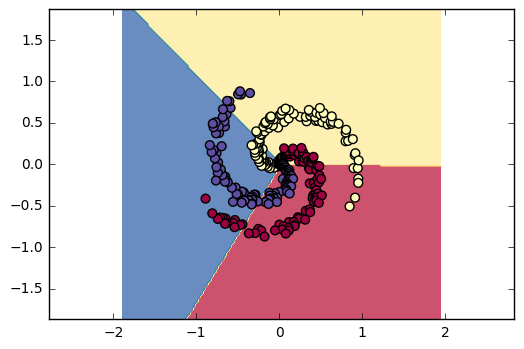The method presented above, kown as (Linear) Logistic regression (because it is linear regression on the log-probabilities), is the baseline tool for classification. It treats class values as labels, and does not use/rely on an arbitrary embedding of the class set in a metric space.

## Training a linear SoftMargin-SVM¶

Another popular strategy: using a Support Vector Machine loss. In its simplest form, the mechanism that computes the scores vector $s$ is linear, just as in the softmax classifier above:

$$s~=~ Wx + b.$$

However, instead of using a probabilistic interpretation, we will focus on geometry and interpret the collection of sets

$$\{ s \geqslant s,s \}, ~~ \{ s \geqslant s,s \}, \{ s \geqslant s,s \}$$

as a partition whose margins should separate the point clouds as well as possible. More precisely, we wish to minimize

$$\text{Cost}_{X,Y}(W,b) ~=~ \sum_{i=1}^I \sum_{k=1}^K 1_{k\neq y_i}\cdot \big( s_i[k] - (s_i[y_i]-\Delta)\big)^+ + \frac{\varepsilon}{2}\,\text{trace}(W^T W)$$

where $s_i = Wx_i + b$, and where $z^+ = \max(0,z)$ denotes the positive part of a real number $z$. This formula penalizes $W$ and $b$ if they assign to "wrong" classes $k \neq y_i$ a score $s_i[k]$ which is nearly or clearly superior to that of the "correct" class $s_i[y_i]$, with a detection margin parameter $\Delta$.

In :
# Compute svm loss, naive implementation with loops.
# We write it in math convention, as it is too esay to get confused otherwise...
def svm_loss_naive(W, X, y, reg):
"""
Structured SVM loss function, naive implementation (with loops)
Inputs:
- W: C x D array of weights
- X: D x N array of data. Data are D-dimensional columns
- y: 1-dimensional array of length N with labels 0...K-1, for K classes
- reg: (float) regularization strength
Returns:
a tuple of:
- loss as single float
- gradient with respect to weights W; an array of same shape as W
"""
dW = np.zeros(W.shape) # initialize the gradient as zero

# compute the loss and the gradient
num_classes = W.shape
num_train   = X.shape
loss = 0.0
for i in range(num_train):
scores              = W.dot(X[:, i]) # vector of size Kx1
correct_class_score = scores[y[i]]   # real number
for j in range(num_classes):
if j == y[i]: # If correct class
continue
# We wish to penalize the model if it gives "wrong" classes
# a score wish is too close to the correct one.
# That is, we wish to minimize the margin
margin = scores[j] - correct_class_score + 1 # (Here, we fix delta=1)
if margin > 0:  # which has a nonzero impact as soon as scores[j] > correct_class_score - 1
loss        += margin
dW[ j,   :] += X[:, i].T
dW[y[i], :] -= X[:, i].T

# Right now the loss is a sum over all training examples, but we want it
# to be an average instead so we divide by num_train.
loss /= num_train
dW   /= num_train                 # Average gradients as well
loss += 0.5 * reg * np.sum(W * W) # Add regularization to the loss.
return loss, dW

In :
# Define vectorized version of svm loss
def svm_loss_vectorized(W, X, y, reg):
"""
Structured SVM loss function, vectorized implementation.
Inputs and outputs are the same as svm_loss_naive.
"""
loss = 0.0
dW = np.zeros(W.shape) # initialize the gradient as zero

scores = np.dot(W, X) # also known as f(x_i, W)

correct_scores = np.ones(scores.shape) * scores[y, np.arange(0, scores.shape)]
deltas = np.ones(scores.shape)
L = scores - correct_scores + deltas

L[L < 0] = 0
L[y, np.arange(0, scores.shape)] = 0 # Don't count y_i
loss = np.sum(L)

# Average over number of training examples
num_train = X.shape
loss /= num_train

loss += 0.5 * reg * np.sum(W * W)

L = scores - correct_scores + deltas

L[L < 0] = 0
L[L > 0] = 1
L[y, np.arange(0, scores.shape)] = 0 # Don't count y_i
L[y, np.arange(0, scores.shape)] = -1 * np.sum(L, axis=0)
dW = np.dot(L, X.T)

# Average over number of training examples
num_train = X.shape
dW /= num_train

return loss, dW

In :
# Define appropriate set-up
W  = 0.01 * np.random.randn(K,D)
XT = X.T

# Check computation time difference between naive and vectorized versions
tic = time.time()
loss_naive, grad_naive = svm_loss_naive(W, XT, y, 0.00001)
toc = time.time()
print('Naive loss: %e computed in %fs' % (loss_naive, toc - tic))

tic = time.time()
loss_vectorized, _ = svm_loss_vectorized(W, XT, y, 0.00001)
toc = time.time()
print('Vectorized loss: %e computed in %fs' % (loss_vectorized, toc - tic))

Naive loss: 1.992376e+00 computed in 0.020579s
Vectorized loss: 1.992376e+00 computed in 0.003211s

In :
# Define classifier training procedure
def train(X, y, step_size, reg, num_iters):
"""
Train this linear classifier using stochastic gradient descent.
Inputs:
- X: D x N array of training data. Each training point is a D-dimensional
column.
- y: 1-dimensional array of length N with labels 0...K-1, for K classes.
- learning_rate: (float) learning rate for optimization.
- reg: (float) regularization strength.
- num_iters: (integer) number of steps to take when optimizing
Outputs:
A list containing the value of the loss function at each training iteration.
"""
dim, num_train = X.shape
num_classes = np.max(y) + 1 # assume y takes values 0...K-1 where K is number of classes

# initialize W
W = np.random.randn(num_classes, dim) * 0.01

# Run gradient descent to optimize W
loss_history = []
for it in range(num_iters):

loss, grad = svm_loss_vectorized(W, X, y, reg)
loss_history.append(loss)

# perform parameter update
W += -1 * step_size * grad

if it % 20 == 0:
print('iteration %d : loss %f' % (it, loss))

return W, loss_history

In :
# Actually compute training
step_size = 1e-0
reg = 1e-3
num_iters = 200
W, loss_history = train(XT,y,step_size,reg,num_iters)

iteration 0 : loss 2.001402
iteration 20 : loss 1.106596
iteration 40 : loss 1.103465
iteration 60 : loss 1.103461
iteration 80 : loss 1.103456
iteration 100 : loss 1.103476
iteration 120 : loss 1.103509
iteration 140 : loss 1.103499
iteration 160 : loss 1.103502
iteration 180 : loss 1.103505

In :
# Compute predictions and training accuracy
scores = np.dot(W, XT)
y_pred = scores.argmax(axis=0)
print('training accuracy: %f' % (np.mean(y == y_pred), ))

training accuracy: 0.520000

In :
# plot the resulting classifier
X = XT.T
W = W.T
h = 0.02
x_min, x_max = X[:, 0].min() - 1, X[:, 0].max() + 1
y_min, y_max = X[:, 1].min() - 1, X[:, 1].max() + 1
xx, yy = np.meshgrid(np.arange(x_min, x_max, h),
np.arange(y_min, y_max, h))
Z = np.dot(np.c_[xx.ravel(), yy.ravel()], W)
Z = np.argmax(Z, axis=1)
Z = Z.reshape(xx.shape)
fig = plt.figure()
plt.contourf(xx, yy, Z, cmap=plt.cm.Spectral, alpha=0.8)
plt.scatter(X[:, 0], X[:, 1], c=y, s=40, cmap=plt.cm.Spectral)
plt.axis("equal") ; plt.xlim(xx.min(), xx.max()) ; plt.ylim(yy.min(), yy.max())

Out:
(-1.8712034092398278, 1.8687965907601756)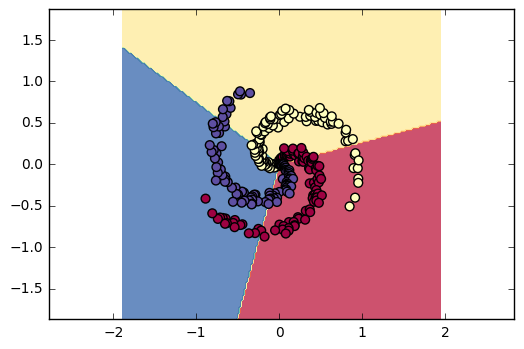## Training SVMs with different kernels¶

Both methods presented above look pretty basic, as they rely on linear scores. Thankfully, we can tweak those linear algorithms to tackle nonlinear problems using the kernel trick. The idea is to embed the data in a higher dimensional feature space, where a linear classifier can be used with more reasonable performance. Thankfully, all of this is done implicitly. Indeed, all the classification algorithms presented above are purely geometric, and can thus be expressed in term of the Gram matrix of the point cloud

$$G_{i,j} ~=~ \langle x_i, y_j \rangle$$

.

Consequently, to work with an embedding $\varphi$, there's no need to be able to actually compute the high-dimensional vectors $\varphi(x)$: having access to the Kernel matrix

$$K_{i,j} ~=~ \langle \varphi(x_i), \varphi(y_j) \rangle ~=~ k(x_i,y_j)$$

is enough. But then, Mercer's theorem gives necessary and sufficient conditions on the kernel function $k$ to be able to interpret the Kernel matrix as a "mapped" Gram matrix. For instance, if we opt for translation invariant kernels $k(x_i,y_j) = k(x_i-y_j)$, they read as

$$\forall~\omega \in \mathbb{R}^D,~~ \widehat{k}(\omega) ~>~ 0$$

,

that is, "the Fourier transform of $k$ should be real and positive". Taking all of this into account, a standard approach to classification is to use SVM or logistic regression (implemented in terms of the Gram matrix), with a custom kernel chosen with respect to the data at hand.

In :
# Define models
C = 1.0  # SVM regularization parameter
models = (svm.SVC(kernel='linear', C=C),
svm.LinearSVC(C=C),
svm.SVC(kernel='poly', degree=3, C=C),
svm.SVC(kernel='rbf', gamma=4., C=C)) # N.B.: "RBF" = "Gaussian kernel"
models = (clf.fit(X, y) for clf in models)

# Define model titles
titles = ('SVC with linear kernel',
'LinearSVC (linear kernel)',
'SVC with polynomial (degree 3) kernel',
'SVC with RBF kernel')

In :
# Plot resulting classifiers
for clf, title in zip(models, titles):
X0, X1 = X[:, 0], X[:, 1]
h = 0.02
x_min, x_max = X[:, 0].min() - 1, X[:, 0].max() + 1
y_min, y_max = X[:, 1].min() - 1, X[:, 1].max() + 1
xx, yy = np.meshgrid(np.arange(x_min, x_max, h),
np.arange(y_min, y_max, h))
Z = clf.predict(np.c_[xx.ravel(), yy.ravel()])
Z = Z.reshape(xx.shape)
fig = plt.figure()
plt.contourf(xx, yy, Z, cmap=plt.cm.Spectral, alpha=0.8)
plt.scatter(X[:, 0], X[:, 1], c=y, s=40, cmap=plt.cm.Spectral)
plt.axis("equal") ; plt.xlim(xx.min(), xx.max()) ; plt.ylim(yy.min(), yy.max())
plt.title(title)
plt.show()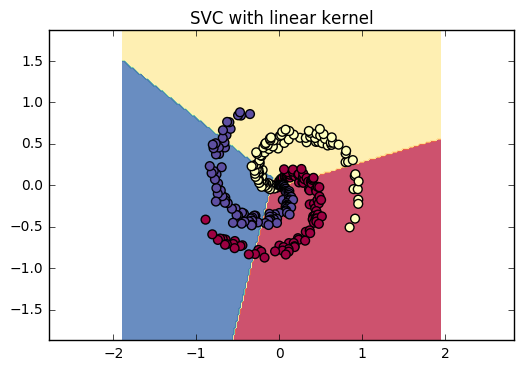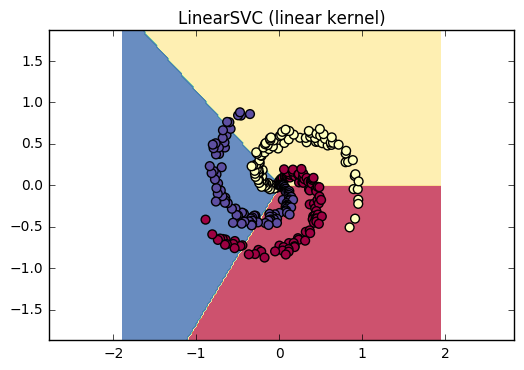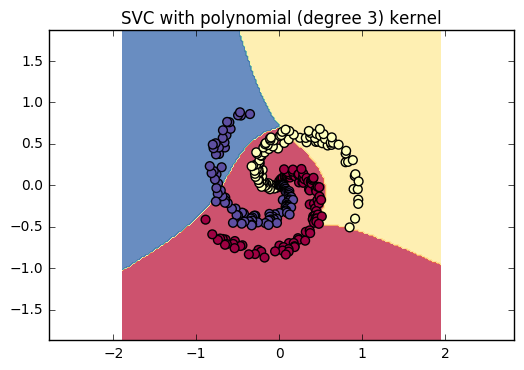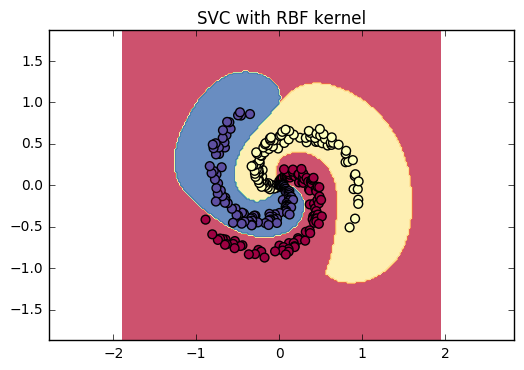As can be seen here, the RBF (Gaussian) kernel SVM pretty much solves the problem for a wide range of values of gamma. Being a robust, well-understood and efficient tool, it is now a standard baseline in the machine learning industry: a popular "next step" after linear/logistic regression.

## Training a 2-layer perceptron¶

After probabilistic models and soft-margin classifiers, a third idea is to try to learn the optimal program for a given classification task. Instead of optimizing in, say, the well-delimited space of decision hyperplanes, the ambition is to search our decision rule $f_w:x_i \mapsto f_w(x_i)\simeq y_i$ in a much wider (and wilder) space of computer programs.

Making a naive tree search in the space of Turing machines / $\lambda$-expressions is obviously intractable: it is a combinatorial problem with crazy complexity - without even speaking of the classical CS paradoxes! In order to link up with efficient optimization routines, it is thus necessary to restrict ourselves to continuously parametrized and finite operators: given elementary computational bricks

$$F^i_{w_i} : \mathbb{R}^{N_{i-1}} \mapsto \mathbb{R}^{N_i}$$

and a fixed program depth $D$, we'll look for the optimal parameters $w=(w_1,\dots,w_D)$ of a classification program

$$f_w ~=~ F^D_{w_D} \circ \cdots \circ F^2_{w_2} \circ F^1_{w_1}.$$

Which kind of elementary block should we use ? From a computational point of view, the simplest choice would be to use linear operators (affine, really). That is, to define $w_i = (W_i, b_i) \in \mathbb{R}^{N_i\times N_{i-1}} \times \mathbb{R}^{N_i}$ and use

$$F^i_{w_i} : x \mapsto W_i x + b_i.$$

Problem is: the composition of two affine functions is still an affine function... That is: concatenating linear operators, increasing the program depth $D$ doesn't help us to explore a wider set of programs. Therefore, the "best" choice will be to "break" the linearity by using atomic operators of the form

$$F^i_{w_i} : x \mapsto \sigma(W_i x + b_i),$$

where $\sigma$ is a pointwise non-linear function; typically, the Rectified Linear Unit, or positive part

$$\sigma : x \mapsto x^+ = \max(0,x).$$

The elementary operators $F^i_{W_i,b_i}$ are both generic and easy to work with. Note that we do not make any sparsity assumption on the matrix $W_i$: a priori, the output coordinates depend of all the input coordinates. In the literature, these elementary bricks are thus known as fully connected layers.

Putting all of this in practice. The next workshop session will be dedicated to this "algorithmic" stance on machine learning, as we investigate its potential for practical applications as well as its limits. For now, let's just see "how it's done" on a simplistic 2D-dataset. We implement a gradient descent search on the parameters of a 2-layer perceptron, that is, of a non-linear operator

$$f_w ~=~ F^2_{W_2,b_2} \circ F^1_{W_1,b_1}$$

where

$$F^1_{W_1,b_1}: x\in \mathbb{R}^2 \mapsto (W_1 x + b_1)^+ \in \mathbb{R}^H ~~~ \text{and} ~~~ F^2_{W_2,b_2}: x\in \mathbb{R}^H \mapsto (W_2 x + b_2) \in \mathbb{R}^K.$$

Here, $H=100$ is the number of "hidden units" in the intermediate vector space $\mathbb{R}^{N_1}$, and $K=3$ is the number of classes. Since $f_w(x_i) \in \mathbb{R}^K$ can be interpreted as a scores vector, we'll use the "softmax+KL" discrepancy discussed in the section on Logistic regression, and interpret the output of our model as a vector of un-normalized log-probabilities. All in all, we strive to minimize

$$\text{Cost}_{X,Y}(W_1,b_1,W_2,b_2) ~=~ - \frac{1}{I}\sum_i \log \bigg( \frac{ \exp(s_i[y_i]) }{\sum_j \exp(s_i[j])} \bigg) + \frac{\varepsilon}{2}\, \text{trace}(W_1^T W_1 + W_2^T W_2)$$

where $s_i ~=~ f_w(x_i)$.

In :
# initialize parameters randomly
H  = 100 # size of hidden layer
W1 = 0.01 * np.random.randn(D,H)
b1 =        np.zeros((1,H))
W2 = 0.01 * np.random.randn(H,K)
b2 =        np.zeros((1,K))

# some hyperparameters
step_size = 1e-0
reg = 1e-3 # regularization strength, "epsilon"

num_examples = X.shape
for i in range(10000):
# evaluate class scores, [N x K]
hidden_layer = np.maximum(0, np.dot(X, W1) + b1) # note, ReLU activation
scores       = np.dot(   hidden_layer, W2) + b2

# compute the class probabilities
exp_scores = np.exp(scores)
probs      = exp_scores / np.sum(exp_scores, axis=1, keepdims=True) # [N x K]

# compute the loss: average cross-entropy loss and regularization
corect_logprobs = -np.log(probs[range(num_examples),y])
data_loss       =  np.sum(corect_logprobs)/num_examples
reg_loss        =  0.5*reg*np.sum(W1*W1) + 0.5*reg*np.sum(W2*W2)
loss            =  data_loss + reg_loss
if i % 1000 == 0:
print("iteration %d: loss %f" % (i, loss))

# compute the gradient on scores
dscores = probs
dscores[range(num_examples),y] -= 1
dscores /= num_examples

# backpropate the gradient to the parameters
# first backprop into parameters W2 and b2
dW2 = np.dot(hidden_layer.T, dscores)
db2 = np.sum(dscores, axis=0, keepdims=True)
# next backprop into hidden layer
dhidden = np.dot(dscores, W2.T)
# backprop the ReLU non-linearity
dhidden[hidden_layer <= 0] = 0
# finally into W,b
dW1 = np.dot(X.T, dhidden)
db1 = np.sum(dhidden, axis=0, keepdims=True)

dW2 += reg * W2
dW1 += reg * W1

# perform a parameter update
W1 += -step_size * dW1
b1 += -step_size * db1
W2 += -step_size * dW2
b2 += -step_size * db2

iteration 0: loss 1.098646
iteration 1000: loss 0.298212
iteration 2000: loss 0.262189
iteration 3000: loss 0.249800
iteration 4000: loss 0.247609
iteration 5000: loss 0.246873
iteration 6000: loss 0.246444
iteration 7000: loss 0.246166
iteration 8000: loss 0.245961
iteration 9000: loss 0.245785

In :
# evaluate training set accuracy
hidden_layer = np.maximum(0, np.dot(X, W1) + b1)
scores = np.dot(hidden_layer, W2) + b2
predicted_class = np.argmax(scores, axis=1)
print('training accuracy: %.2f' % (np.mean(predicted_class == y)))

training accuracy: 0.98

In :
# plot the resulting classifier
h = 0.02
x_min, x_max = X[:, 0].min() - 1, X[:, 0].max() + 1
y_min, y_max = X[:, 1].min() - 1, X[:, 1].max() + 1
xx, yy = np.meshgrid(np.arange(x_min, x_max, h),
np.arange(y_min, y_max, h))
Z = np.dot(np.maximum(0, np.dot(np.c_[xx.ravel(), yy.ravel()], W1) + b1), W2) + b2
Z = np.argmax(Z, axis=1)
Z = Z.reshape(xx.shape)
fig = plt.figure()
plt.contourf(xx, yy, Z, cmap=plt.cm.Spectral, alpha=0.8)
plt.scatter(X[:, 0], X[:, 1], c=y, s=40, cmap=plt.cm.Spectral)
plt.axis("equal") ; plt.xlim(xx.min(), xx.max()) ; plt.ylim(yy.min(), yy.max())

Out:
(-1.8712034092398278, 1.8687965907601756)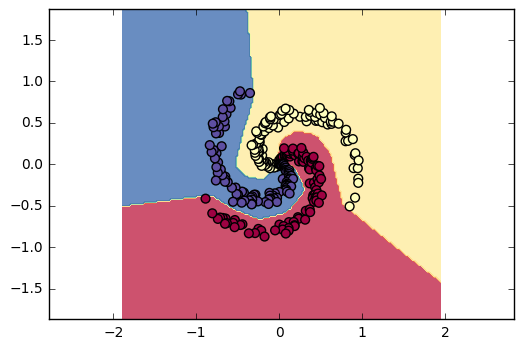Since the 60's (one layer) and 80's (multilayer), the models presented above were known as a "multilayer perceptrons". As it is not a very relatable name, and since these stacked data flows are (very) loosely related to some structures discovered in the cortex of mammals, these programs $f_w = F^D\circ \cdots\circ F^1$ are now colloquially referred to as "neural networks". In the next workshop session, we will present extensions of these ideas to higher dimensional problems (esp. image classification) which have been recently marketed as "Deep Learning" in the mass media.

## Conclusion¶

Today, we've presented a few strategies to fit "trainable models" $f_w$ to a supervised dataset $(x_i,y_i)$. Interestingly, even though these algorithms are very close to each other (a one-layer perceptron is basically logistic regression...), they can be interpreted from very different angles! In just one Notebook, we've introduced:

• Probabilistic modeling: Logistic regression (of which we've only scratched the surface), Gaussian mixture models...
• Geometric classification: SVMs, k-means++...
• Algorithmic regression: Perceptrons, CNNs, RNNs...

Today, we asserted visually the relevance of our classifiers. But as soon as the dimensionality of the data points exceeds 3, this becomes intractable! The usual safeguard is to split the dataset into a training set, used by the optimization loop, and a test set used to compute a final classification score. If both subsets are independent (and if one does not fine-tune the hyper-parameters too much), this protocol allows us to get an independent measure of the quality of our trained model.

How can machine learning algorithms generalize on unobserved data? The data scientist's fiercest enemy is overfit: when a model has memorized the training set well, without actually learning any generalizable decision rule. This can for instance happen if the trained model $f_w$ converges towards the "hash table" function

$$f_w \longrightarrow \sum_{i\in\text{Training}} y_i\cdot 1_{\{x_i\}}.$$

To prevent this from happening, the key ingredient is the regularization prior encoded in the algorithm. Even for linear models, things are not as simple as they may seem: As the classifier is constrained to being linear, it should not be able to learn a gibberish decision rule... Except if the dimensionality of the input vector $x$ is too high! On images for instance, a linear classifier can easily overfit on the value of a single pixel or on any other meaningless decision rule, if it happens to "separate" the training set well enough. Quantifying the strength of the regularization prior (compared to, say, the signal to noise ratio and the number of training samples) is a difficult task, and still an open problem for neural networks. In the algorithms above, the amount of regularization is encoded in the following elements:

• Linear Logistic regression/SVM: dimensionality of the input space + the explicit $L^2$ regularization prior on the linear scores matrix $W$. The latter may seem innocuous; but remember that if the canonical basis used to encode your data does not make sense with respect to your problem, neither does the $L^2$ norm! You could, at the very least, think of replacing it with another euclidean metric on the space of matrices.
• Kernel methods: intrinsic dimensionality of the dataset with respect to the kernel used. For instance, a large kernel width "blurs" the dataset and reduces the risk of overfit.
• Neural networks: implicit combination of the model architecture + the $L^2$ (stochastic) gradient descent scheme used to optimize it.